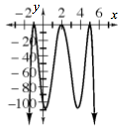### Home > A2C > Chapter 10 > Lesson 10.2.6 > Problem10-146

10-146.
1. Make a sketch of the graph of each of the following functions. Describe the graphs. Homework Help ✎

1. y = (x + 1)2(x − 2)2(x − 5)2

2. y = −(x + 1)2(x − 2)2(x − 5)2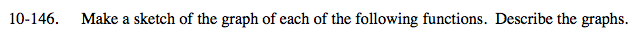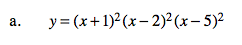What are the roots?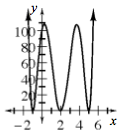Look closely at the equation and graph, what are the x-intercepts?

Double roots at −1, 2, 5.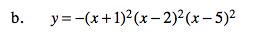See part (a).